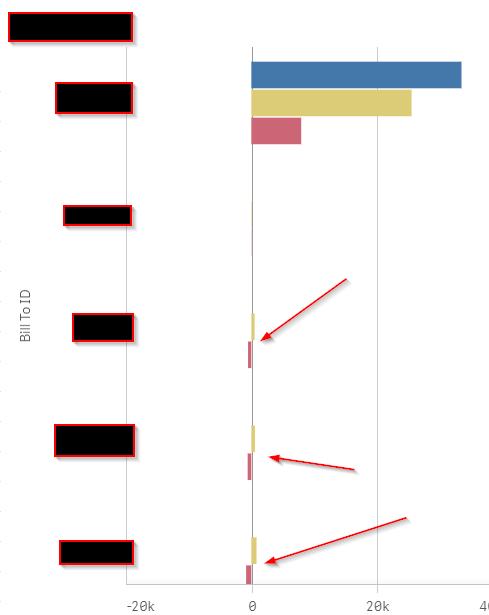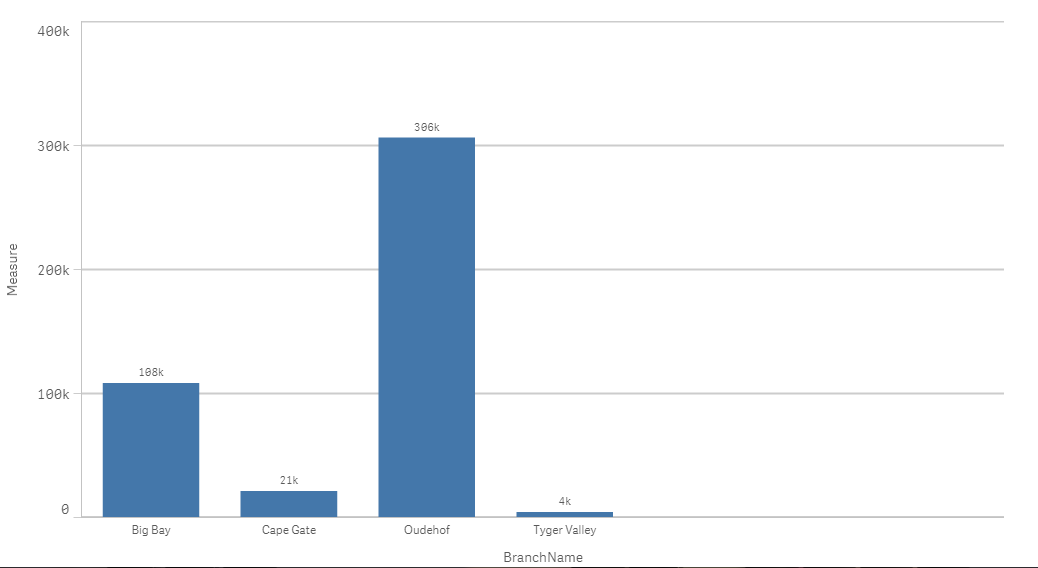# New to Qlik Sense

Discussion board where members can get started with Qlik Sense.

Announcements
Qlik® Product Spotlight: Discover what’s possible. Get more from our products.
See for yourself. Register today.
Not applicable

## Filter Chart using Aggregate function

Hello Folks,

I have a chart where I compute the aggregate sum for a particular field.  When the value of this field is Equal To or Less Than 0, I don't want this data point to appear in the bar chart.  However, when I put the aggregate function in my Dimension > Field I get "Invalid Dimension"

Below is a picture with arrows pointing to data points that I want hidden.This the aggregate function I am using to determine if a data point should appear or not.

if(

Sum({<Year = {\$(=Max(Year))}>} ChickenWings) - Sum({<Year = {\$(=Max(Year-1))}>} ChickenWings) > 0,

[Bill To ID], Null()

)

Any help is greatly appreciated.

1 Solution

Accepted Solutions
Not applicable

## Re: Filter Chart using Aggregate function

This way?First thing, uncheck this:and change your expression as follow:

if((SUM({<Year={\$(=Max(Year))}>}Sales) - SUM({<Year={\$(=Max(Year)-1)}>}Sales))>0,

(SUM({<Year={\$(=Max(Year))}>}Sales) - SUM({<Year={\$(=Max(Year)-1)}>}Sales)),Null())

see the attached app

5 Replies
Not applicable

## Re: Filter Chart using Aggregate function

Have you tried to uncheck show null values under complement? If that does not solve it;
Can you please share a sample QVF file?

Not applicable

## Re: Filter Chart using Aggregate function

Hello Omar,

I've attached a file to this message.  An example would be the Head Office, who year-over-year has a negative sales value.  In this case, I don't want 'Head Office' to appear in the chart.

Any help is greatly appreciated.

Not applicable

## Re: Filter Chart using Aggregate function

This way?First thing, uncheck this:and change your expression as follow:

if((SUM({<Year={\$(=Max(Year))}>}Sales) - SUM({<Year={\$(=Max(Year)-1)}>}Sales))>0,

(SUM({<Year={\$(=Max(Year))}>}Sales) - SUM({<Year={\$(=Max(Year)-1)}>}Sales)),Null())

see the attached app

Not applicable

## Re: Filter Chart using Aggregate function

One last question, what if I have a bar chart with multiple bars and I want to hide the value if one of the Measures is negative.

Not applicable

## Re: Filter Chart using Aggregate function

Same logic with  each measure:

measure1 : if(expression1>0,expression1,null())

measure2 : if(expression2>0,expression2,null())

measure3 : if(expression3>0,expression3,null())

Community Browser